Science, Maths & Technology

### Become an OU studentGeometry

Start this free course now. Just create an account and sign in. Enrol and complete the course for a free statement of participation or digital badge if available.

# 2.9 Similar and congruent shapes

Two shapes are said to be similar if they are the same shape but not necessarily the same size. In other words, one may be an enlargement of the other. They may also have different orientations, as in the drawing below.

When a photograph is enlarged, the two images are similar.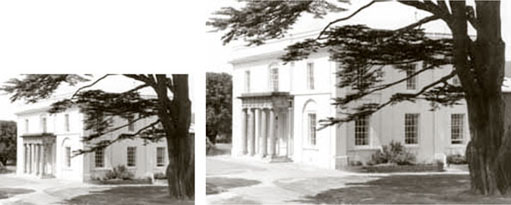But if a photograph is stretched in only one direction, the resulting shape is not similar to the original.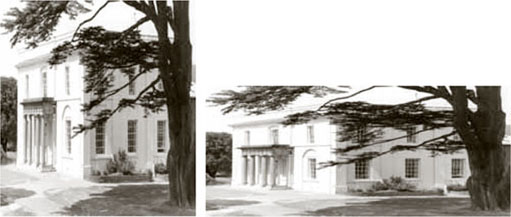In effect, when two shapes are similar, one is a scaled up (or down) version of the other. Thus an accurate model and its original will be similar in this mathematical sense. If you measure the sides of the model, you will find that to produce the original, each side must be scaled up by the same amount. However, the angles remain the same in each version.

The simplest scaled shapes are similar triangles. In two similar triangles, angles in equivalent positions must be the same size. This provides a way of identifying similar triangles.

It is not necessary to calculate all the angles in two similar triangles. If two angles in one triangle match two angles in the other, then the third angle must also be the same in both, because in each case it will be 180° minus the sum of the other two angles.

Examples of similar triangles are set out below.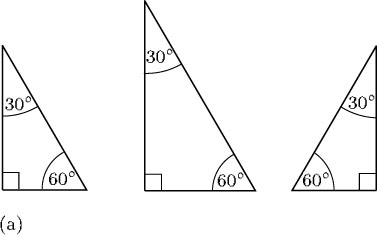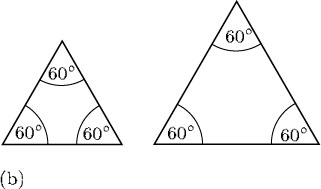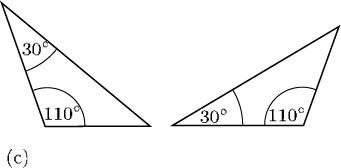If two figures are the same shape and the same size, they are said to be congruent.

## Example 10

This diagram shows, in simplified form, a wooden buttress supporting the wall of a medieval church.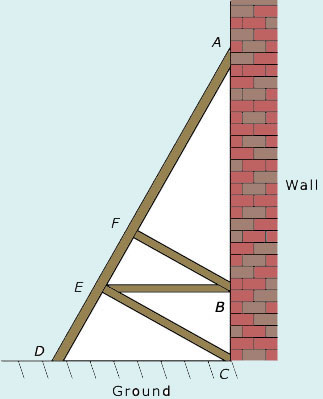The angle between the ground and the buttress,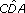, is 65°. By making appropriate assumptions, identify which triangles are similar. Calculate all the angles in the structure.

Assume that the wall is vertical, and that the ground and BE are both horizontal. Also assume that BF and CE are at right angles to AD.

Consider the angles of ΔACD: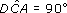and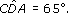Then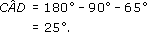It is easiest to see which triangles are similar if you look at them in pairs.

In each diagram, the two triangles under consideration are emphasised by heavy lines.

In (a), both the triangles that are outlined by heavy lines have the same angle at A, 25°, and both also have a right angle (at C and B, respectively). Therefore the third angle in the two triangles (at D and E) must also be the same. (You can confirm this by noticing that these are corresponding angles.) The size of these angles must be 180° − 25° − 90° = 65°.

In (b), both triangles have the same angle at A, 25°, and they both have a right angle (at E and F, respectively). Then the third angle in each will be the same size, 65°.

In (c), each triangle has a right angle (at E and F, respectively), and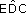in the larger triangle is the same size as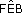in the smaller triangle (they are corresponding angles). These corresponding angles are each 65°; hence the third angle must again be 25°.

This gives six triangles, each with angles of 25°, 90° and 65°, and so all are similar.

There is a seventh triangle that is also similar to the others, ΔBEC. This has a right angle, and its angle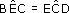in ΔECD (they are alternate angles), and so is 25°. Its third angle must therefore be 65°.

You may have met other examples of similar shapes, for example, when using scale diagrams. The scale plan of a house is similar to the actual layout of the house.